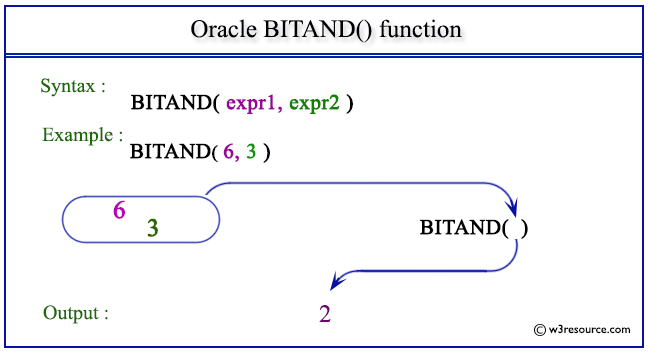# Oracle BITAND() function

## Description

This BITAND() function returns the output as bitwise AND of the inputs, the inputs, and its output are treats as vectors of bits.
The types of inputs are the number, and the result is of type number. If either argument to BITAND is NULL, the result is NULL.
The arguments must be in the range -(2(n-1)) .. ((2(n-1))-1). If an argument is out of this range, the result is undefined.

Syntax:

`BITAND(expr1, expr2)`

Arguments:

Name Description
expr1 A number.
expr2 A number.

The types of expr1 and expr2 are NUMBER, and the result is of type NUMBER.

Pictorial Presentation of BITAND() functionExample:

``````SELECT BITAND(6,3) FROM dual;
```
```

Here is the result.

```BITAND(6,3)
-----------
2
```

Previous: ATAN2
Next: CEIL

﻿